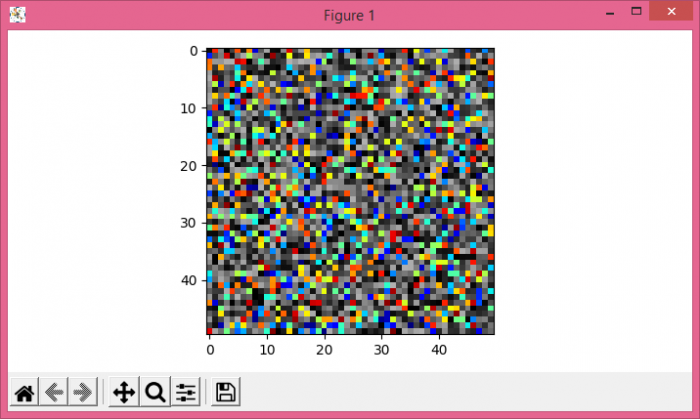# Setting Transparency Based on Pixel Values in Matplotlib

To set transparency based on pixel values in matplotlib, get masked data wherever data is less than certain values. Lesser value will result in full overlapping between two images.

## Steps

• Create data1 and data2 using numpy.

• Using subplots() method, create a figure and a set of subplots (fig and ax).

• Display the data (data1 and masked data) as an image, i.e., on a 2D regular raster, using imshow() method, with different colormaps, jet and gray.

• To display the figure, use show() method.

## Example

import numpy as np
import matplotlib.pyplot as plt
import matplotlib.cm as cm
plt.rcParams["figure.figsize"] = [7.00, 3.50]
plt.rcParams["figure.autolayout"] = True
data1 = np.random.rand(50, 50)
data2 = np.random.rand(50, 50)
plt.show()